﻿ 主动配电网中分布式电源最大接入容量分析 Study on the Maximum Penetration of Active Distribution Network

Advances in Energy and Power Engineering
Vol.05 No.05(2017), Article ID:22535,9 pages
10.12677/AEPE.2017.55017

Study on the Maximum Penetration of Active Distribution Network

Kang Qian1, Ling Wei2, Shouzhen Zhu2, Jinghong Zheng2

2Department of Electrical Engineering, Tsinghua University, Beijing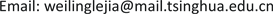Received: Oct. 5th, 2017; accepted: Oct. 19th, 2017; published: Oct. 31st, 2017ABSTRACT

One of the significant features of the active distribution network is the access to a large number of distributed generations. In the new situation, the efficient use of distributed generations needs to study the distributed generations’ impact on active distribution network. In this paper, the steady-state voltage distribution of the discrete load is deduced to the steady-state voltage distribution in the general continuous analytical form by using the steady-state constant power load model and the distributed generation model. By analyzing the characteristics and requirements of the steady-state voltage distribution, the maximum penetration of the distributed generations under the condition of no voltage limit is analyzed. The influence of the line regulator on the voltage distribution after the distributed generations is also analyzed.

Keywords:Active Distribution Network, Distributed Generation, Maximum Penetration, Steady-State Voltage Distribution

1江苏电力设计院有限公司，江苏 南京

2清华大学电机系，北京1. 引言

2. 稳态电压分布

2.1. 分布式电源和负荷模型

2.2. 考虑离散负荷时的稳态电压分布

1) 两节点简单接线

${U}_{1}\approx \left({U}_{0}+\Delta {U}_{1}\right)={U}_{0}-\frac{P\cdot R+Q\cdot X}{{U}_{0}}=\mathrm{Re}\left({\overline{U}}_{1}\right)$ (1)

$\Delta {U}_{1}={{U}^{\prime }}_{1}-{U}_{1}=\mathrm{Re}\left({\overline{{U}^{\prime }}}_{1}\right)-\mathrm{Re}\left({\overline{U}}_{1}\right)=\frac{{P}_{dg}\cdot R+{Q}_{dg}\cdot X}{{U}_{0}}$ (2)

2) 多节点链式网络Figure 1. Two nodes with DGFigure 2. Multi-node chain feeder

$\Delta {U}_{n}=\Delta {U}_{n\to 0}={\sum }_{l=1}^{n}\Delta {U}_{l\to l-1}$ (3)

$e{U}_{n}=\Delta {{U}^{\prime }}_{n}-\Delta {U}_{n}=\frac{1}{{U}_{0}}\left\{\begin{array}{l}{P}_{dg}{\sum }_{i=1}^{n}\text{ }{R}_{i}+{Q}_{dg}{\sum }_{i=1}^{n}\text{ }{X}_{i}\text{\hspace{0.17em}}\text{\hspace{0.17em}}\text{\hspace{0.17em}}\text{\hspace{0.17em}}1\le n (4)

${U}_{n}={U}_{0}-\Delta {U}_{n}$ (5)

2.3. 考虑连续负荷时电压分布的解析分析

$\Delta {U}^{\prime }\left(l\right)=\left\{\begin{array}{l}{\int }_{0}^{l}\left(i\left(l\right)-{I}_{dg}\right)Z\text{d}l\text{\hspace{0.17em}}\text{\hspace{0.17em}}\text{\hspace{0.17em}}\text{\hspace{0.17em}}\text{\hspace{0.17em}}\text{\hspace{0.17em}}\text{\hspace{0.17em}}\text{\hspace{0.17em}}\text{\hspace{0.17em}}\text{\hspace{0.17em}}\text{\hspace{0.17em}}\text{\hspace{0.17em}}\text{\hspace{0.17em}}\text{\hspace{0.17em}}\text{\hspace{0.17em}}\text{\hspace{0.17em}}\text{\hspace{0.17em}}\text{\hspace{0.17em}}\text{\hspace{0.17em}}\text{\hspace{0.17em}}\text{\hspace{0.17em}}\text{\hspace{0.17em}}\text{\hspace{0.17em}}\text{\hspace{0.17em}}\text{\hspace{0.17em}}\text{\hspace{0.17em}}\text{\hspace{0.17em}}\text{\hspace{0.17em}}0 (6)

$\Delta {U}^{\prime }\left(l\right)=\left\{\begin{array}{l}{\int }_{0}^{l}\left(i\left(l\right)-{\sum }_{mk=1}^{M}{I}_{dg,mk}\right)Z\text{d}l\text{\hspace{0.17em}}\text{\hspace{0.17em}}\text{\hspace{0.17em}}\text{\hspace{0.17em}}\text{\hspace{0.17em}}\text{\hspace{0.17em}}\text{\hspace{0.17em}}\text{\hspace{0.17em}}\text{\hspace{0.17em}}\text{\hspace{0.17em}}\text{\hspace{0.17em}}\text{\hspace{0.17em}}\text{\hspace{0.17em}}\text{\hspace{0.17em}}\text{\hspace{0.17em}}\text{\hspace{0.17em}}\text{\hspace{0.17em}}\text{\hspace{0.17em}}\text{\hspace{0.17em}}\text{\hspace{0.17em}}\text{\hspace{0.17em}}\text{\hspace{0.17em}}\text{\hspace{0.17em}}\text{\hspace{0.17em}}\text{\hspace{0.17em}}\text{\hspace{0.17em}}\text{\hspace{0.17em}}\text{\hspace{0.17em}}\text{\hspace{0.17em}}\text{\hspace{0.17em}}\text{\hspace{0.17em}}\text{\hspace{0.17em}}\text{\hspace{0.17em}}\text{\hspace{0.17em}}\text{\hspace{0.17em}}\text{\hspace{0.17em}}\text{\hspace{0.17em}}\text{\hspace{0.17em}}\text{\hspace{0.17em}}\text{\hspace{0.17em}}\text{\hspace{0.17em}}\text{\hspace{0.17em}}\text{\hspace{0.17em}}\text{\hspace{0.17em}}\text{\hspace{0.17em}}\text{\hspace{0.17em}}\text{\hspace{0.17em}}\text{\hspace{0.17em}}\text{\hspace{0.17em}}\text{\hspace{0.17em}}\text{\hspace{0.17em}}\text{\hspace{0.17em}}\text{\hspace{0.17em}}\text{\hspace{0.17em}}\text{\hspace{0.17em}}\text{\hspace{0.17em}}\text{\hspace{0.17em}}\text{\hspace{0.17em}}\text{\hspace{0.17em}}\text{\hspace{0.17em}}\text{\hspace{0.17em}}\text{\hspace{0.17em}}\text{\hspace{0.17em}}\text{\hspace{0.17em}}\text{\hspace{0.17em}}\text{\hspace{0.17em}}\text{\hspace{0.17em}}\text{\hspace{0.17em}}\text{\hspace{0.17em}}\text{\hspace{0.17em}}\text{\hspace{0.17em}}\text{\hspace{0.17em}}\text{\hspace{0.17em}}\text{\hspace{0.17em}}\text{\hspace{0.17em}}\text{\hspace{0.17em}}\text{\hspace{0.17em}}\text{\hspace{0.17em}}{L}_{dg0} (7)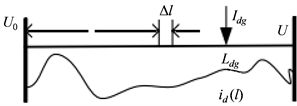Figure 3. Feeder model with continuous distributed load

$U\left(l\right)={U}_{0}-{\int }_{0}^{l}\left(i\left(l\right)-{I}_{dg}\left(l\right)\right)Z\text{d}l={U}_{0}-{\int }_{0}^{l}\left[{\int }_{l}^{L}\left({i}_{d}\left(\tau \right)-{i}_{dgd}\left(\tau \right)\right)\text{d}\tau \right]Z\text{d}l$ (8)

2.4. 线路调压器的影响

2.5. 分布式电源最大可接入容量的研究

2.5.1. 分布式电源可行接入位置

$\frac{\text{d}}{\text{d}l}U\left(l\right)=\left\{\begin{array}{l}-\left(i\left(l\right)-{I}_{dg}\right)Z\text{\hspace{0.17em}}\text{\hspace{0.17em}}\text{\hspace{0.17em}}\text{\hspace{0.17em}}\text{\hspace{0.17em}}\text{\hspace{0.17em}}\text{\hspace{0.17em}}0 (9)

$i\left({l}^{*}\right)={I}_{dg}$ (10)

$\left(i\left({L}_{dg}^{*}\right)-{I}_{dg}\right)Z=-i\left({L}_{dg}^{*}\right)Z$ (11)

$i\left({L}_{dg}^{*}\right)=\frac{1}{2}{I}_{dg}$ (12)

2.5.2. 分布式电源最大接入容量

DG的接入位置确定以后，注入功率是需要考虑的另一个关键问题。考察 $\left[{l}^{\text{*}},{L}_{dg}\right]$ 之间电压的上升区，如果方程 $\Delta U\left(l\right)=0$ 有解 ${l}_{0}$ ，则说明在 ${l}_{0}$ 处电压与送端电压相同。保证该方程有解的必要条件是 ${l}^{\text{*}}<{L}_{dg}$ ，且解 ${l}_{0}$ 必然满足不等式 ${l}^{*}<{l}_{0}<{L}_{dg}$

${L}_{dg}{I}_{dg}\le {\int }_{0}^{{L}_{dg}}i\left(l\right)\text{d}l$ (13)

${I}_{dg}^{*}=\frac{{\int }_{0}^{{L}_{dg}}i\left(l\right)\text{d}l}{{L}_{dg}}$ (14)

${U}_{0}-{\int }_{0}^{{L}_{dg}}\left(i\left(l\right)-{I}_{dg\mathrm{max}}\right)Z\text{d}l\le {U}_{\mathrm{max}}$ (15)

3. 仿真算例

3.1. 算例条件

3.2. 算例分析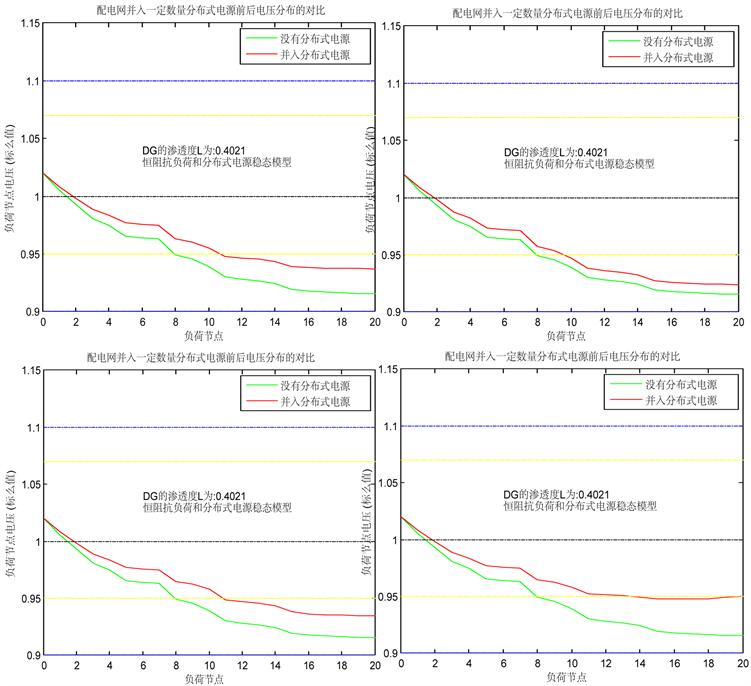Figure 4. Voltage profile curves with different DG locations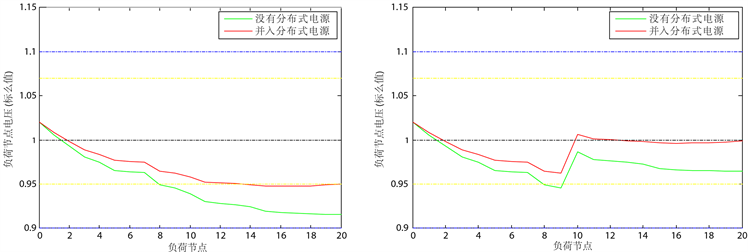Figure 5. Voltage profile curves of DG with line regulator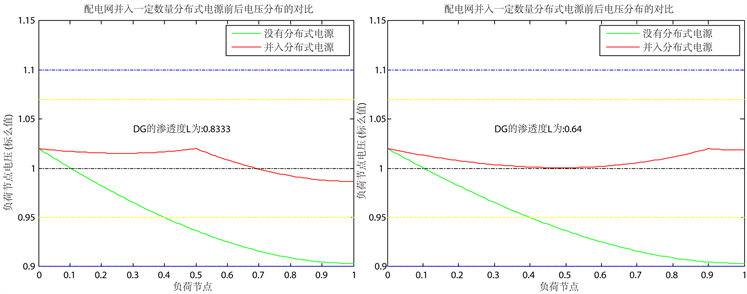Figure 6. Voltage profile curves of max penetration of DG with different locationsTable 2. Max penetration of DG with different locations under voltage limit

${i}_{d}\left(l\right)=0.5+l$ (16)

4. 结论

Study on the Maximum Penetration of Active Distribution Network[J]. 电力与能源进展, 2017, 05(05): 106-114. http://dx.doi.org/10.12677/AEPE.2017.55017

1. 1. 黄炜, 刘健. 分布式光伏电源极端可接入容量极限研究[J]. 电力系统保护与控制, 2015, 43(2): 22-28.

2. 2. 陈祥鹏. 光伏发电并网对配电网电压分布影响的研究[D]: [硕士学位论文]. 淄博: 山东理工大学, 2015.

3. 3. 刘健, 同向前. 考虑过电压因素时分布式光伏电源的准入容量[J]. 电力系统保护与控制, 2014, 42(6): 45-51.

4. 4. 夏成军, 崔弘. 考虑静态安全约束的分布式电源准入容量计算[J]. 电网技术, 2009, 33(16): 96-100.

5. 5. 苏小玲, 韩民晓. 配电网中分布式电源最大准入容量分析[J]. 电网技术, 2012, 36(10): 87-92.

6. 6. 阎鼎, 包海龙. 配电网中分布式电源最大准入容量优化配置[J]. 2015, 27(10): 16-20.

7. 7. 刘科研, 刘永梅. 考虑电压约束的分布式电源接入配电网最大准入容量计算方法[J]. 电力自动化设备, 2016, 36(6): 81-86.

8. 8. 王磊, 夏昊君. 考虑分布式电源最佳接入位置的最大准入容量研究[J]. 中国电力设备工业, 2016(2): 74-78.

9. 9. 刘慧珍, 巩晓昕. 北京电网多元分布式电源的准入容量计算[J]. 电气应用, 2015(s1): 211-215.

10. 10. 王志群, 朱守真, 周双喜. 分布式发电接入位置和注入容量限制的研究[J]. 电力系统自动化, 2005, 17(1): 53-58.